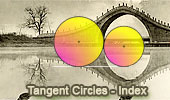Online Geometry: Tangent Circles, Theorems and Problems Page 3

 Tangent Circles, Theorems and Problems: Table of Content 3/8Proposed Problem 418. Triangle, Incircle, Inradius, Equal Tangent circles, Radius. Proposed Problem 417. Tangent circles, Tangent lines, Angles. Proposed Problem 403. Circular sector, 90 degrees, Circle, Semicircle, Area. Proposed Problem 394. Square, 90 Degree Arc, Diagonal, Congruence. Proposed Problem 393. Triangle, Orthocenter, Circumcircles, Congruence, Collinear. Proposed Problem 370. Triangle with squares, Circumcircles, Tangent circles. Proposed Problem 336. Two equal circles, a Common Tangent and a Square. Proposed Problem 333. Circle inscribed in a semicircle, Perpendicular to the common tangent. Go to page: Previous | 1 | 2 | 3 | 4 | 5 | 6 | 7 | 8 | Next